﻿ ToposPro » Computing and analyzing the migration map in α-AgI
Computing and analyzing the migration map in α-AgI

Algorithm:
1. Open the database electrolytes, and choose AgI/alpha. Compute the adjacency matrix Analyze the structure in IsoCryst. Determine the number of iodine atoms closest to a silver atom. For this purpose, select an Ag atom, leave it on the plot only, toggle to the mode and apply the tool to draw all atoms in the sphere of a given radius (2.85 Å) (see the value at the bottom bar).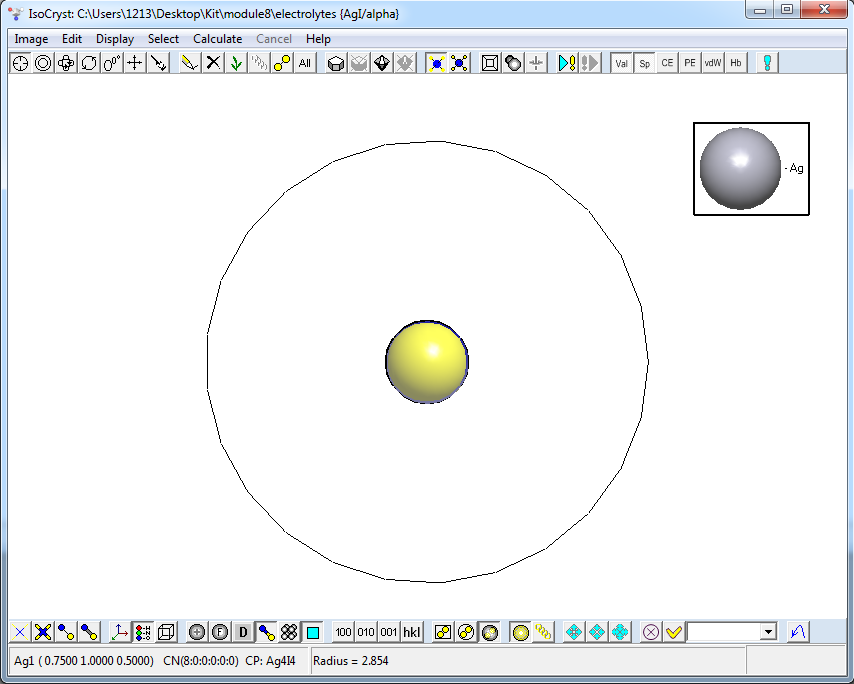Be sure that the Ag atom is allocated in a tetrahedral void of four I atoms.

2.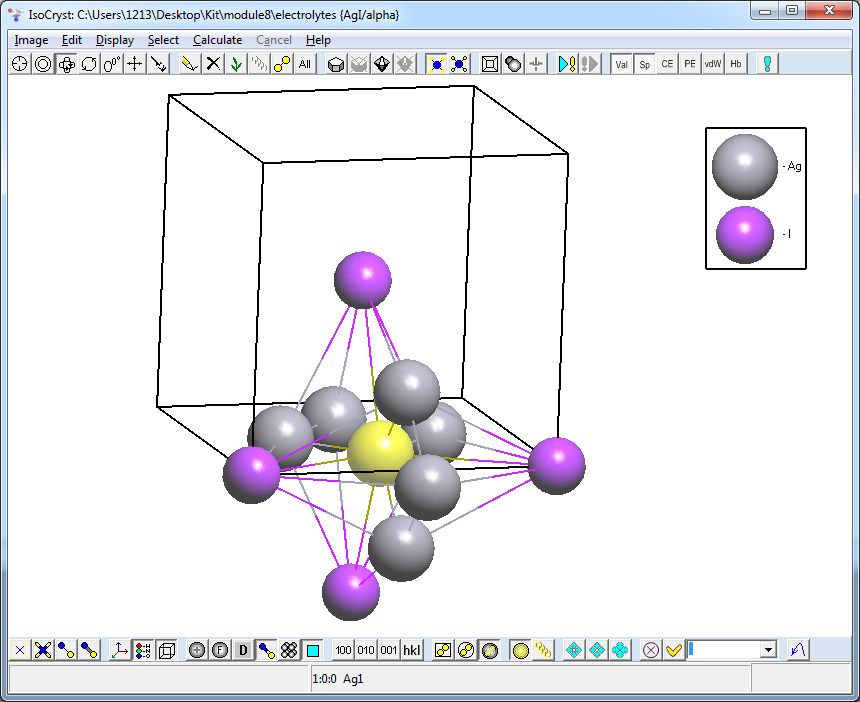3. Build the system of voids and channels in the sublattice of iodine atoms ignoring Ag atoms. For this purpose, open a Dirichlet window and specify the following options in the Continuous tab: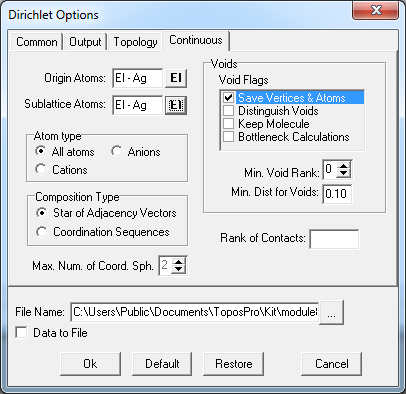Run Dirichlet. You will get the new electrolytes# database where the data on the initial structure as well as the data on voids and channels are stored.

4. Draw the structure from the electrolytes# database in IsoCryst. Remove Ag atoms. Be sure that their positions correspond to the positions of void centers, and that the system of channels in the structure is 3-periodic (the Ag red atoms superimpose the blue ZA voids).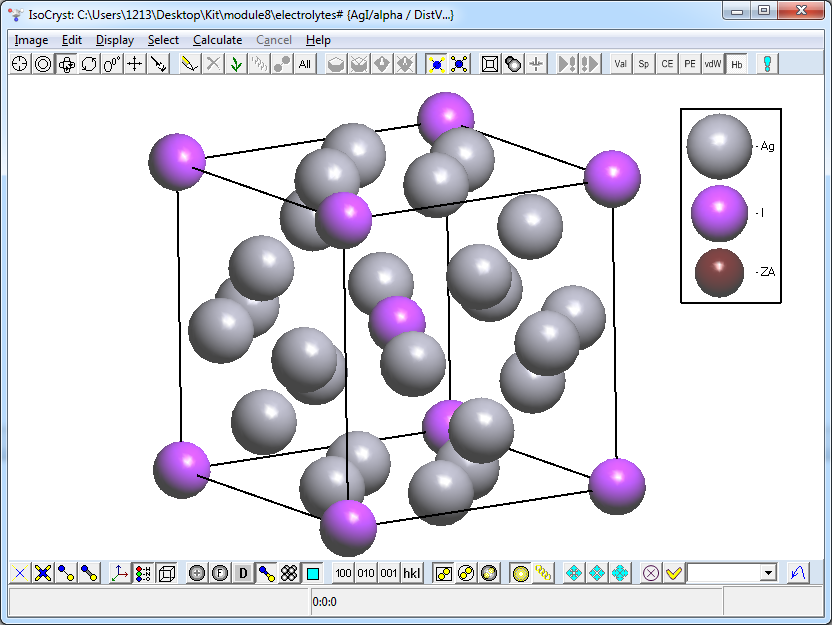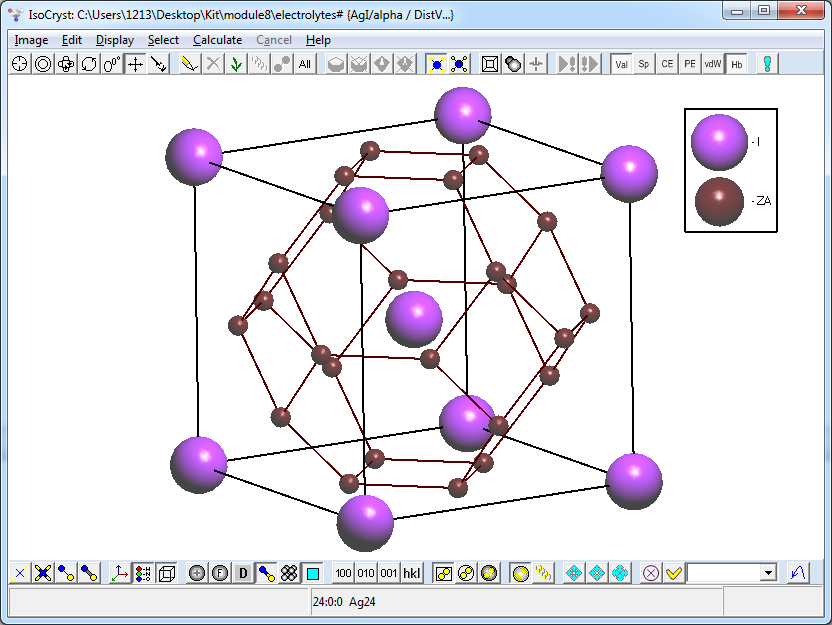5. Analyze the geometric parameters of voids and channels. For this purpose, open a Crystal Data window and go to the Adjacency Matrix tab. Extend the list of channels spreading from the void. Find the radius of the void (Rsd) and radii of the channels (Rad).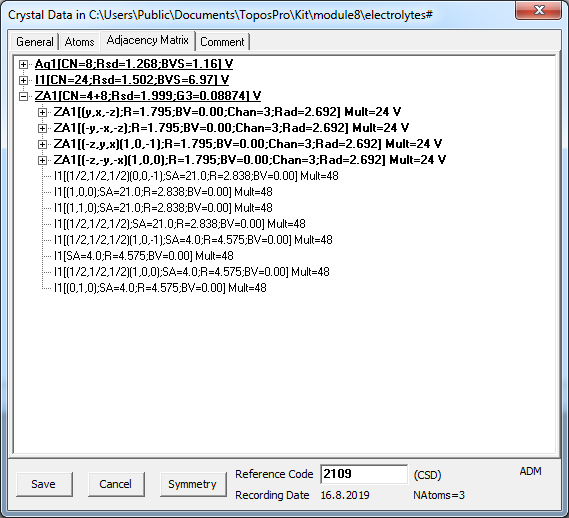Compare the channels radius (2.692 Å) with the sum (3.0 Å) of radii of Ag and I. The values of Slater’s radii for the atoms can be found in IsoCryst options (Atoms/Radii) Ag=1.600 Å, I=1.400 Å.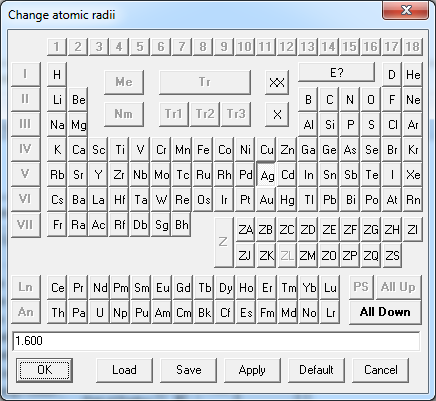The channel is assumed to be accessible for mobile ions if the sum of radii of the mobile ion and the framework atom exceeds the channel radius no more than by 10-15%. Are the channels accessible for Ag+ ions? Yes, the channels are accessible to Ag+ ions, because the radius of the channel (2.692 Å) is greater than the sum of radii of Ag and I minus 15%: (3.0 - 3.0⋅0.15) = 2.55 Å, but smaller that 10% threshold (3-0.3 = 2.7 Å). 2.55 Å < 2.692 Å < 2.7 Å so it is likely that the conductivity is possible at high temperature.